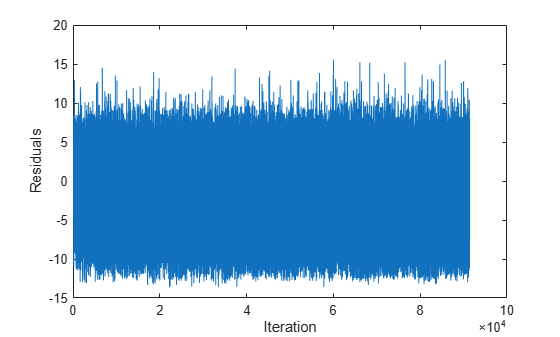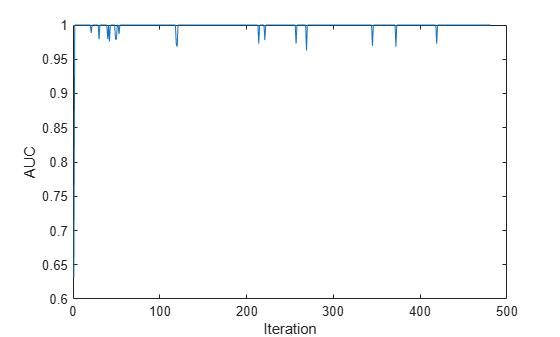# predict

Predict responses for new observations from linear incremental learning model

Since R2020b

## Syntax

``label = predict(Mdl,X)``
``label = predict(Mdl,X,'ObservationsIn',dimension)``
``[label,score] = predict(___)``

## Description

example

````label = predict(Mdl,X)` returns the predicted responses (or labels) `label` of the observations in the predictor data `X` from the incremental learning model `Mdl`.```

example

``label = predict(Mdl,X,'ObservationsIn',dimension)` specifies the observation dimension of the predictor data, either `'rows'` (default) or `'columns'`. For example, specify `'ObservationsIn','columns'` to indicate that observations in the predictor data are oriented along the columns of `X`.`

example

``[label,score] = predict(___)` also returns classification scores for all classes when `Mdl` is an incremental learning model for classification, using any of the input argument combinations in the previous syntaxes.`

## Examples

collapse all

Load the human activity data set.

`load humanactivity`

For details on the data set, enter `Description` at the command line.

Responses can be one of five classes: Sitting, Standing, Walking, Running, or Dancing. Dichotomize the response by identifying whether the subject is moving (`actid` > 2).

`Y = actid > 2;`

Fit a linear classification model to the entire data set.

`TTMdl = fitclinear(feat,Y)`
```TTMdl = ClassificationLinear ResponseName: 'Y' ClassNames: [0 1] ScoreTransform: 'none' Beta: [60x1 double] Bias: -0.2005 Lambda: 4.1537e-05 Learner: 'svm' Properties, Methods ```

`TTMdl` is a `ClassificationLinear` model object representing a traditionally trained linear classification model.

Convert the traditionally trained linear classification model to a binary classification linear model for incremental learning.

`IncrementalMdl = incrementalLearner(TTMdl)`
```IncrementalMdl = incrementalClassificationLinear IsWarm: 1 Metrics: [1x2 table] ClassNames: [0 1] ScoreTransform: 'none' Beta: [60x1 double] Bias: -0.2005 Learner: 'svm' Properties, Methods ```

`IncrementalMdl` is an `incrementalClassificationLinear` model object prepared for incremental learning using SVM.

• The `incrementalLearner` function initializes the incremental learner by passing learned coefficients to it, along with other information `TTMdl` learned from the training data.

• `IncrementalMdl` is warm (`IsWarm` is `1`), which means that incremental learning functions can start tracking performance metrics.

• The `incrementalLearner` configures the model to be trained using the adaptive scale-invariant solver, whereas `fitclinear` trained `TTMdl` using the BFGS solver

An incremental learner created from converting a traditionally trained model can generate predictions without further processing.

Predict class labels for all observations using both models.

```ttlabels = predict(TTMdl,feat); illables = predict(IncrementalMdl,feat); sameLabels = sum(ttlabels ~= illables) == 0```
```sameLabels = logical 1 ```

Both models predict the same labels for each observation.

If you orient the observations along the columns of the predictor data matrix, you can experience an efficiency boost during incremental learning.

Load and shuffle the 2015 NYC housing data set. For more details on the data, see NYC Open Data.

```load NYCHousing2015 rng(1) % For reproducibility n = size(NYCHousing2015,1); shuffidx = randsample(n,n); NYCHousing2015 = NYCHousing2015(shuffidx,:);```

Extract the response variable `SALEPRICE` from the table. Apply the log transform to `SALEPRICE`.

```Y = log(NYCHousing2015.SALEPRICE + 1); % Add 1 to avoid log of 0 NYCHousing2015.SALEPRICE = [];```

Create dummy variable matrices from the categorical predictors.

```catvars = ["BOROUGH" "BUILDINGCLASSCATEGORY" "NEIGHBORHOOD"]; dumvarstbl = varfun(@(x)dummyvar(categorical(x)),NYCHousing2015,... 'InputVariables',catvars); dumvarmat = table2array(dumvarstbl); NYCHousing2015(:,catvars) = [];```

Treat all other numeric variables in the table as linear predictors of sales price. Concatenate the matrix of dummy variables to the rest of the predictor data, and transpose the data to speed up computations.

```idxnum = varfun(@isnumeric,NYCHousing2015,'OutputFormat','uniform'); X = [dumvarmat NYCHousing2015{:,idxnum}]';```

Configure a linear regression model for incremental learning with no estimation period.

`Mdl = incrementalRegressionLinear('Learner','leastsquares','EstimationPeriod',0);`

`Mdl` is an `incrementalRegressionLinear` model object.

Perform incremental learning and prediction by following this procedure for each iteration:

• Simulate a data stream by processing a chunk of 100 observations at a time.

• Fit the model to the incoming chunk of data. Specify that the observations are oriented along the columns of the data. Overwrite the previous incremental model with the new model.

• Predict responses using the fitted model and the incoming chunk of data. Specify that the observations are oriented along the columns of the data.

```% Preallocation numObsPerChunk = 100; n = numel(Y); nchunk = floor(n/numObsPerChunk); r = nan(n,1); figure h = plot(r); h.YDataSource = 'r'; ylabel('Residuals') xlabel('Iteration') % Incremental fitting for j = 2:nchunk ibegin = min(n,numObsPerChunk*(j-1) + 1); iend = min(n,numObsPerChunk*j); idx = ibegin:iend; Mdl = fit(Mdl,X(:,idx),Y(idx),'ObservationsIn','columns'); yhat = predict(Mdl,X(:,idx),'ObservationsIn','columns'); r(idx) = Y(idx) - yhat; refreshdata drawnow end````Mdl` is an `incrementalRegressionLinear` model object trained on all the data in the stream.

The residuals appear symmetrically spread around 0 throughout incremental learning.

To compute posterior class probabilities, specify a logistic regression incremental learner.

Load the human activity data set. Randomly shuffle the data.

```load humanactivity n = numel(actid); rng(10); % For reproducibility idx = randsample(n,n); X = feat(idx,:); Y = actid(idx);```

For details on the data set, enter `Description` at the command line.

Responses can be one of five classes: Sitting, Standing, Walking, Running, or Dancing. Dichotomize the response by identifying whether the subject is moving (`actid` > 2).

`Y = Y > 2;`

Create an incremental logistic regression model for binary classification. Prepare it for `predict` by specifying the class names and arbitrary coefficient and bias values.

```p = size(X,2); Beta = randn(p,1); Bias = randn(1); Mdl = incrementalClassificationLinear('Learner','logistic','Beta',Beta,... 'Bias',Bias,'ClassNames',unique(Y));```

`Mdl` is an `incrementalClassificationLinear` model. All its properties are read-only. Instead of specifying arbitrary values, you can take either of these actions to prepare the model:

• Train a logistic regression model for binary classification using `fitclinear` on a subset of the data (if available), and then convert the model to an incremental learner by using `incrementalLearner`.

• Incrementally fit `Mdl` to data by using `fit`.

Simulate a data stream, and perform the following actions on each incoming chunk of 50 observations.

1. Call `predict` to predict classification scores for the observations in the incoming chunk of data. The classification scores are posterior class probabilities for logistic regression learners.

2. Call `rocmetrics` to compute the area under the ROC curve (AUC) using the incoming chunk of data, and store the result.

3. Call `fit` to fit the model to the incoming chunk. Overwrite the previous incremental model with a new one fitted to the incoming observations.

```numObsPerChunk = 50; nchunk = floor(n/numObsPerChunk); auc = zeros(nchunk,1); % Incremental learning for j = 1:nchunk ibegin = min(n,numObsPerChunk*(j-1) + 1); iend = min(n,numObsPerChunk*j); idx = ibegin:iend; [~,posteriorProb] = predict(Mdl,X(idx,:)); rocObj = rocmetrics(Y(idx),posteriorProb,Mdl.ClassNames); auc(j) = rocObj.AUC(1); Mdl = fit(Mdl,X(idx,:),Y(idx)); end```

`Mdl` is an `incrementalClassificationLinear` model object trained on all the data in the stream.

Plot the AUC on the incoming chunks of data.

```plot(auc) ylabel('AUC') xlabel('Iteration')```The plot suggests that the classifier predicts moving subjects well during incremental learning.

## Input Arguments

collapse all

Incremental learning model, specified as an `incrementalClassificationLinear` or `incrementalRegressionLinear` model object. You can create `Mdl` directly or by converting a supported, traditionally trained machine learning model using the `incrementalLearner` function. For more details, see the corresponding reference page.

You must configure `Mdl` to predict labels for a batch of observations.

• If `Mdl` is a converted, traditionally trained model, you can predict labels without any modifications.

• Otherwise, `Mdl` must satisfy the following criteria, which you can specify directly or by fitting `Mdl` to data using `fit` or `updateMetricsAndFit`.

• If `Mdl` is an `incrementalRegressionLinear` model, its model coefficients `Mdl.Beta` and bias `Mdl.Bias` must be nonempty arrays.

• If `Mdl` is an `incrementalClassificationLinear` model, its model coefficients `Mdl.Beta` and bias `Mdl.Bias` must be nonempty arrays and the class names in `Mdl.ClassNames` must contain two classes.

• Regardless of object type, if you configure the model so that functions standardize predictor data, the predictor means `Mdl.Mu` and standard deviations `Mdl.Sigma` must be nonempty arrays.

Batch of predictor data for which to predict labels, specified as a floating-point matrix of n observations and `Mdl.NumPredictors` predictor variables. The value of `dimension` determines the orientation of the variables and observations.

Note

`predict` supports only floating-point input predictor data. If your input data includes categorical data, you must prepare an encoded version of the categorical data. Use `dummyvar` to convert each categorical variable to a numeric matrix of dummy variables. Then, concatenate all dummy variable matrices and any other numeric predictors. For more details, see Dummy Variables.

Data Types: `single` | `double`

Predictor data observation dimension, specified as `'columns'` or `'rows'`.

Example: `'ObservationsIn','columns'`

Data Types: `char` | `string`

## Output Arguments

collapse all

Predicted responses (labels), returned as a categorical or character array; floating-point, logical, or string vector; or cell array of character vectors with n rows. n is the number of observations in `X`, and `label(j)` is the predicted response for observation `j`.

• For regression problems, `label` is a floating-point vector.

• For classification problems, `label` has the same data type as the class names stored in `Mdl.ClassNames`. (The software treats string arrays as cell arrays of character vectors.)

The `predict` function classifies an observation into the class yielding the highest score. For an observation with `NaN` scores, the function classifies the observation into the majority class, which makes up the largest proportion of the training labels.

Classification scores, returned as an n-by-2 floating-point matrix when `Mdl` is an `incrementalClassificationLinear` model. n is the number of observations in `X`. `score(j,k)` is the score for classifying observation `j` into class `k`. `Mdl.ClassNames` specifies the order of the classes.

If `Mdl.Learner` is `'svm'`, `predict` returns raw classification scores. If `Mdl.Learner` is `'logistic'`, classification scores are posterior probabilities.

collapse all

### Classification Score

For linear incremental learning models for binary classification, the raw classification score for classifying the observation x, a row vector, into the positive class is

`$f\left(x\right)={\beta }_{0}+x\beta ,$`

where

• β0 is the scalar bias `Mdl.Bias`.

• β is the column vector of coefficients `Mdl.Beta`.

The raw classification score for classifying x into the negative class is –f(x). The software classifies observations into the class that yields the positive score.

If the linear classification model consists of logistic regression learners, then the software applies the `'logit'` score transformation to the raw classification scores.

## Version History

Introduced in R2020b